Surface Area: Rectangles  |  Cylinders      Volume: Rectangles  |  Cylinders

# Math Resources Grade 6 Alberta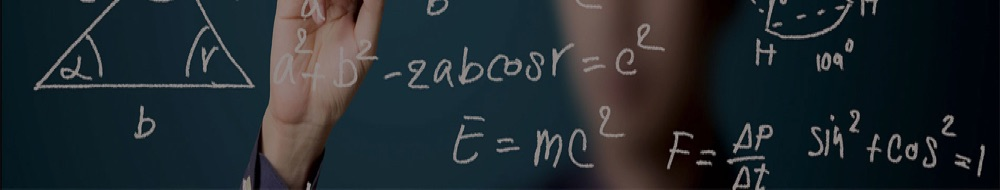MATHEMATICS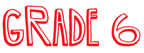Are you working on your math but can’t remember what that ‘annoying’ math word means? Use this Math Dictionary, organized alphabetically, to help you figure it out. Crisis averted!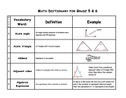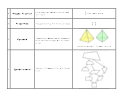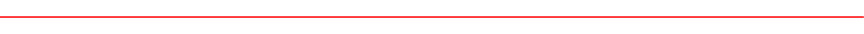Having numbers in our world is very important.  Numbers are constantly used in real life situations.  Many real life examples can involve dealing with stocks, calculating taxes or counting your money when saving up for the snowboard you’ve always wanted.  In grade six, we strive to teach our students the many uses of numbers.  We have been working on strategies for solving operational problems involving addition, subtraction, Multiplication and Division. The students are encouraged to try different strategies that will make it easier for them to solve their basic facts.

Understanding Numbers

DecimalsFractions and decimals are everywhere in our lives. You use them all the time and often don’t even realize it. Cooking, money, sports, time, games, and medicine are just a few examples where you use fractions or decimals. As part of this unit, we will be working with these ideas to improve our number sense skills. When did you use fractions last?

MathsIsFun.com [Explanations, videos and resources for all concepts]

Explanations:   Fractions  |  Decimals        Extra Practise:   Fractions  |  Decimals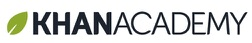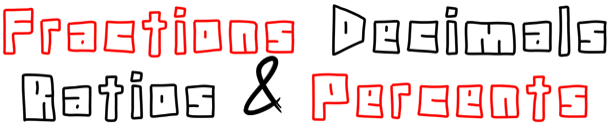Students will be completing these questions as we move through the unit: [Fractions Ratios & Percents Questions]What are the odds the Flames will make the playoffs? How likely is it for the Blue Jays to beat the Yankees? The Red Sox? If the students in the class were asked what their favourite sport was, how many would say hockey? These are all questions that could be used in our investigation into Statistics & Probability.

Students will be completing these questions as we move through the unit: [Stats & Probability Questions]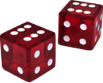Angles & Polygons Review Questions

Geometry & Measurement Review Questions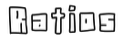Our world is filled with shapes large and small, with angles, lines and different geometric shapes. It’s the oldest math, the most basic math - “What shapes fit together to let me build something?” In this unit, students will be exploring the world of angles, polygons, volume and area, as well as how we can measure these. Lastly, students will be exploring 2D and 3D shapes with their different characteristics and learning how to transform (move) them around coordinate grids.

Measurement Resources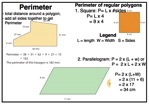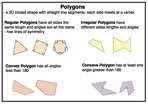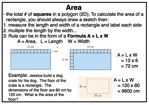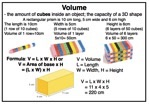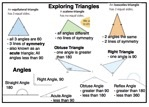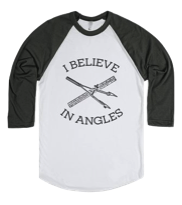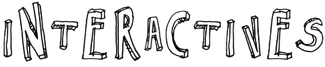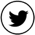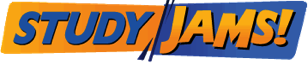Probability

StatisticsMeasurement

Geometry